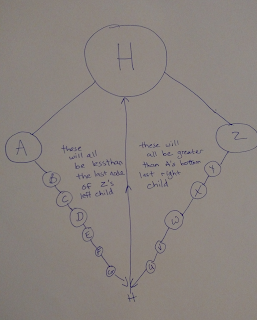## Posts

Showing posts from February, 2019

### refactor Python Binary TreeI have an older post where I posted this code, but I wanted to explain my 'delete' method in more detail.

Take a look at this: [alt attribute attached]

This depicts how at some point, depending on which side of a node you are on, there is a limit to how far a branch can reach.
'A' would be the Left child node of 'H', and the farthest it's right branches can reach is to 'H'.   Anything more than H, is on the other child node on the right.

Likewise, for child node 'Z',  it's left most branches have the same limit of 'H'.  Because all other smaller/lesser than keys would go on the left child node.

At first I had my delete method throwing all right children onto the right branch of the left child  and replacing the parent node with that modified left child.
But then I realized: [alt in image]

The right most branch of the right side of root has limitless potential.  It is not restricted in any way by the median for how long/large it …

### pandas python and probability Part 2So I may have a really weird way going about figuring this stuff out.
I pound in code, break it tons of times and then keep googling until I find what I need to fix each break.

I have a very strong feeling the test first crowd would be furious with me....

But,  here it goes.

I wanted to create a training set for my machine learning experiment.
This is the order in which I did that:
1) create the pandas database
pd.set_option('max_columns', 100)
train_data = { 'run number': , #'run number:' as key produces NaN results
'odd probability': [0.50],
'even probability': [0.50],
}
train_prob_data = pd.DataFrame(train_data, columns = ['run number', 'odd
probability', 'even probability'])
2) create a list of random numbers

def create_data():
datalist = []
for coin_toss in range(100):
rand_num = randint(1, 20)
…

### Pandas Python and a little probabilityOk, so after looking through different tutorials, I found out pandas is way easier to handle then SQL.

So for the first step in that machine learning experiment from the last post, I came up with this little piece of code.
It uses random/randomint and pandas as import.
Resources and references are in the code comments.
As always, drop me a comment, hate it, love it, what to improve.
Next step is to get this rolling with more math involved and a bigger sets of probability data to store.
Adding an 'old_odd_probability' and 'old_even_probability' would probably be the next step toward getting that bayes to interpret a bigger picture of when and how random chooses it's *random integers*.

For example, to use the water droplet example from Jurassic Park (assuming you repeat the experiment 5 times)...

https://scienceonblog.wordpress.com/2017/04/13/chaos-theory-in-jurassic-park/

If the droplet tends to roll left off your hand the second time you try, and you do it the sam…